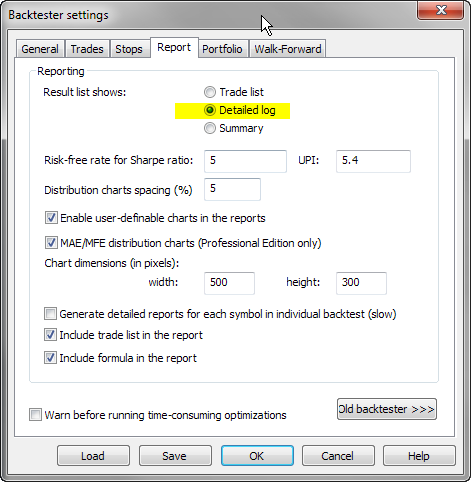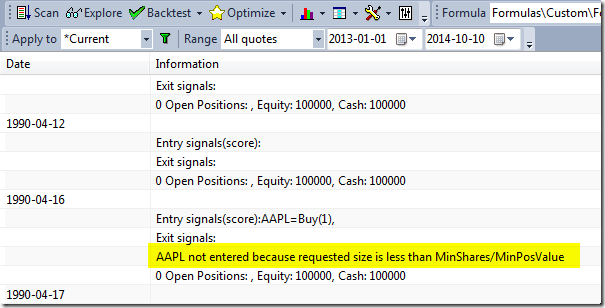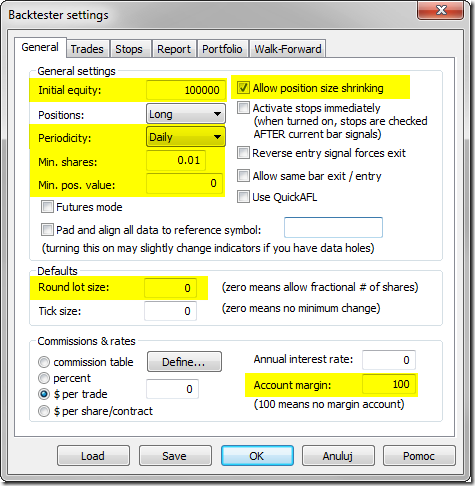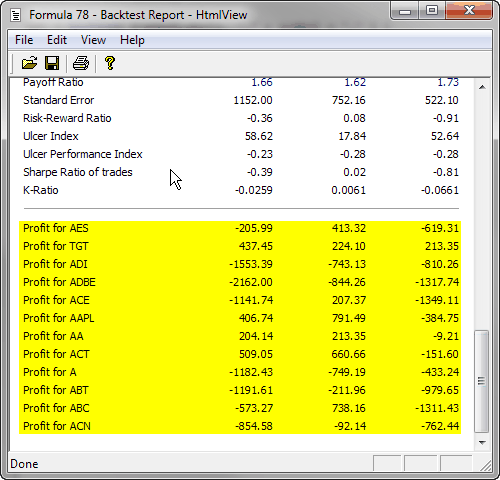#amibroker

### How to restrict trading to certain hours of the day

In order to include time-based conditions in the back-testing code – we can use TimeNum() function to check the time-stamp of given bar and use it as input for any time-based conditions.
http://www.amibroker.com/f?timenum

In order to code a strategy that triggers trades only in certain hours of the day, 9:30-11:00 in this example, we can use the following approach (code uses simple MACD crossovers to generate signals):

`tn = TimeNum();startTime = 93000; // start in HHMMSS formatendTime = 110000;  // end in HHMMSS formattimeOK = tn >= startTime AND tn <= endTime;Buy = Cross( MACD(), Signal() ) AND timeOK;Sell = Cross( Signal(), MACD() ) AND timeOK; `

It is also possible to force an exit signal after 11:00 to avoid overnight positions:

`tn = TimeNum();startTime = 93000; // start in HHMMSS formatendTime = 110000;  // end in HHMMSS formattimeOK = tn >= startTime AND tn <= endTime;Buy = Cross( MACD(), Signal() ) AND timeOK;Sell = (Cross( Signal(), MACD() ) AND timeOK) OR Cross( tn, endTime ); `

### How to synchronize backtesting setup on different computers

When comparing the output of back-tests obtained from different working machines, it is necessary to make sure that all aspects of our testing are identical, including:

1. the database
2. the formula used for testing
3. the settings

In order to synchronize data – the best is to copy the entire local database folder. Using just the same data source, especially if it is real-time feed may not be enough due to different array lengths or some corrections that may have been applied in historical data on data-vendors server in between.

In case of any differences in results between two computers that is the very fist thing to check, as different input would result in different output.

To find out that the data are different you may simply create a checksum of data columns, using code like shown below:

`Filter = Status("lastbarinrange");AddColumn( Cum( High + Low + Close + Open ), "Price checksum");AddColumn( Cum( Volume ), "Volume checksum" );AddColumn( BarIndex(), "Number of bars");AddSummaryRows( 1 ); // add total sum of column`

By running this code on both computers you can compare checksums to see if they are the same.

In order to transfer the formula and settings to the other machine it is enough to:

1. select Analysis window
2. use File->Save from the main menu and save the APX file
3. open the same APX file on the other computer

If data, code and settings are identical, then the obtained results will also stay in sync.

### Handling limit orders in the backtester

In order to simulate limit orders in backtesting it is necessary to check in the code if Low price of the entry bar is below the limit price we want to use. The following example shows an entry signal based on Close price crossing over 100-period simple moving average. The position is opened on the next bar if price drops 1% below the Close of signal bar.

`BuySignal = Cross( Close, MA(Close, 100 ) );// buy on the next barBuy = Ref( BuySignal, -1);BuyLimitPrice = Ref( Close, -1) * 0.99;// now we check if limit was hitBuy = Buy AND L < BuyLimitPrice;// if Open price is below the limit, then we use Open for entryBuyPrice = Min( Open, BuyLimitPrice )`

If we want the order to be valid for more than one bar, then we can use Hold function for this purpose:

`BuySignal = Cross( Close, MA(Close, 100 ) );// buy on the next barBuy = Ref( BuySignal, -1);BuyLimitPrice = ValueWhen(BuySignal, Close) * 0.99;// now we check if limit was hitBuy = Hold( Buy, 3 ) AND L < BuyLimitPrice;// if Open price is below the limit, then we use Open for entryBuyPrice = Min( Open, BuyLimitPrice )`

In a portfolio-level backtest we usually advocate against using limit orders. Why? Simply because we may not have enough cash in your account to place limit orders for all possible entry candidates. If your trading system generates 100 possible entries, you would need to place 100 limit orders only to find out that eventually only few of them fired. With limited buying power, we may need to place limit orders only for the top N-scored tickers that have generated BuySignal and skip the others. To simulate the situation when we only place small set of limit orders for top ranked stocks we can use new ranking functionalities introduced in AmiBroker 5.70. Knowing the rank at this stage is required if we only want to allow orders for top-scored tickers. Let us say that we prefer symbols with smallest RSI values.

The code would look the following way: Formula first generates a ranking for all tickers included in the test (below example uses Watchlist 0), then when testing individual symbols – checks the pre-calculated rank and generates Buy signal based on that reading.

`// we run the code on WatchList 0List = CategoryGetSymbols( categoryWatchlist, 0 );SetOption("MaxOpenPositions", 3);if ( Status("stocknum") == 0 ) // Generate ranking when we are on the very first symbol{     StaticVarRemove( "values*" );     for ( n = 0; ( Symbol = StrExtract( List, n ) )  != "";  n++    )     {         SetForeign ( symbol );         // value used for scoring         values = 100 - RSI();         RestorePriceArrays();         StaticVarSet (  "values"  +  symbol, values );         _TRACE( symbol );     }     StaticVarGenerateRanks( "rank", "values", 0, 1224 );}symbol = Name();values = StaticVarGet ( "values" +  symbol );rank = StaticVarGet ( "rankvalues" +  symbol );PositionScore = values;BuySignal = Cross( Close, MA(Close, 100 ) );// buy on the next barBuy = Ref( BuySignal, -1);BuyLimitPrice = Ref( Close, -1) * 0.999;// now we check if limit was hit for the symbols ranked as top 3Buy = Buy AND L < BuyLimitPrice AND rank <= 3;BuyPrice = Min( Open, BuyLimitPrice );// sample exit rules - 5 - bar stopSell = 0;ApplyStop( stopTypeNBar, stopModeBars, 5, 1)`

Detailed description of the ranking functionality used above is available in the manual at: http://www.amibroker.com/guide/h_ranking.html

### How to display indicator values in the backtest trade list

Backtesting engine in AmiBroker allows to add custom metrics to the report, both in the summary report and in the trade list. This is possible with Custom Backtester Interface, which allows to modify the execution of portfolio-level phase of the test and (among many other features) adjust report generation.

Due to the fact that the report generation occurs in 2nd phase of the test, when the backtester works on ~~~EQUITY ticker, we can not refer directly to given indicators. For example, to display ATR values – calling ATR() function directly is not enough, because we want to see ATR values of the traded symbol, while in portfolio-phase of the test we are no longer working on that symbol’s quotes.

So, we need to:

1. store the values of indicators in static variables in the 1st phase of the test (when individual symbols are processed). This can be done with static variables, creating separate static variable for each symbol
2. read stored values once the backtester reaches the portfolio phase of the test.

The following formula shows how this can be coded. The formula below displays the value of ATR indicator for the entry bar of given trade:

`SetCustomBacktestProc( "" );if ( Status( "action" ) == actionPortfolio ){    bo = GetBacktesterObject();    // run default backtest procedure without generating the trade list    bo.Backtest( True );    // iterate through closed trades    for ( trade = bo.GetFirstTrade( ); trade; trade = bo.GetNextTrade( ) )    {        // read ATR values and display as custom metric        symbolATR = StaticVarGet( trade.Symbol + "ATR" );        trade.AddCustomMetric( "Entry ATR", Lookup( symbolATR, trade.EntryDateTime ) );    }    // iterate through open positions    for ( trade = bo.GetFirstOpenPos( ); trade; trade = bo.GetNextOpenPos( ) )    {        // read ATR values and display as custom metric        symbolATR = StaticVarGet( trade.Symbol + "ATR" );        trade.AddCustomMetric( "Entry ATR", Lookup( symbolATR, trade.EntryDateTime ) );    }    // generate trade list    bo.ListTrades( );}// your trading system hereBuy = Cross( MACD(), Signal() );Sell = Cross( Signal(), MACD() );// assign indicator values to ticker-specific variablesStaticVarSet( Name() + "ATR", ATR( 15 ) )`

### Troubleshooting procedure when backtest shows no trades

When we run backtest and get no results at all – there may be several reasons of such behaviour. The main potential causes are the following:

1. our system does not generate any entry signals within the tested range
2. our settings do not allow the backtester to take any trades

To verify if we are getting any signals – the first thing to do is to run a Scan. This allows us to check if we are getting any Buy or Short signals at all. If there are none, then we need to check the formula and make sure that data interval we are working on are correct (in Periodicity in Analysis->Settings->General).

If Scan works fine and returns trading signals, but backtester still does not produce any output, it usually means that the settings are wrong, i.e. the constraints set in the settings prevent trades from being opened mainly because requested position size is too big or too small.

To check what is going on, it is best to switch Report mode to Detailed log and re-run backtest.Once you run backtest in Detailed Log mode you will be able to find out exact reasons why trades can not be opened for each and every bar:Using the following settings may be helpful to minimize chances of not entering trades because of various constraints:

In Analysis->Settings, General tab:

1. check if Initial Equity is high enough
2. set Periodicity to the appropriate interval
3. Allow position size shrinking – turn it On
4. Round Lot Size – set it to 0
5. in Min. Shares box enter 0.01
6. in Min. pos. value enter 0
7. Account Margin – set it to 100in Portfolio tab, enter 0 in Limit trade size as % of entry bar volume box.### How to exclude top ranked symbol(s) in rotational backtest

Rotational trading is based on scoring and ranking of multiple symbols based on user-defined criteria. For each symbol a user-definable “score” is assigned on bar by bar basis. Then, each bar, symbols are sorted according to that score and N top ranked symbols are bought, while existing positions that don’t appear in top N rank are closed.

Sometimes however, we may want to exclude the highest ranking symbol (or a couple of them) from trading. The code below shows how to do that using custom backtester.

`ExcludeTopN = 1; // how many top positions to excludeSetCustomBacktestProc("");if ( Status( "action" ) == actionPortfolio ){    bo = GetBacktesterObject();    bo.PreProcess();    for ( bar = 0; bar < BarCount; bar++ )    {        Cnt = 0;        for ( sig = bo.GetFirstSignal( bar ); sig; sig = bo.GetNextSignal( bar ) )        {            if ( Cnt < ExcludeTopN )                sig.Price = -1; // exclude            Cnt++;        }        bo.ProcessTradeSignals( bar );    }    bo.PostProcess();}EnableRotationalTrading( True );SetOption( "MaxOpenPositions", 5 );SetOption( "WorstRankHeld", 10 );SetPositionSize( 20, spsPercentOfEquity );PositionScore = 1 / RSI( 14 )`

The code is pretty straightforward mid-level custom backtest loop but it uses one trick – setting signal price to -1 tells AmiBroker to exclude given signal from further processing. Note also that signals retrieved by GetFirstSignal / GetNextSignal are already sorted, so the highest ranked signal appears first in the list.

### Using price levels with ApplyStop function

ApplyStop function by default requires us to provide stop amount (expressed in either dollar or percentage distance from entry price). Therefore, if we want to place stop at certain price level, then we need to calculate the corresponding stop amount in our code.

This example shows how to place stops at previous bar Low (for long trades) and previous bar High (for short trades).

Stop amount parameter is simply the distance between entry price and desired trigger price (exit point). For long trade it is entry price minus stop level, while for short trade it is trigger (exit) price minus entry price. Additionally we may check if calculated distance is at least 1-tick large. We can distinguish between long and short entry by checking if one of entry signals is present (if a Buy signal is active then it is long entry, otherwise short). We only need to take care about the fact that if we are using trade delays we need to get delayed Buy signal as shown in the code below:

`TradeDelay = 1; // set it to 0 for no delaysSetTradeDelays( TradeDelay, TradeDelay, TradeDelay, TradeDelay );TickSize = 0.01;// sample entry rulesBuy = Cross( MACD(), Signal() );Short = Cross( Signal(), MACD() );Sell = Cover = 0; // no other exit conditions, just stopsBuyPrice = SellPrice = ShortPrice = CoverPrice = Close;// define stop levelstopLevelLong = Ref( L, -1 ); // use previous bar lowstopLevelShort = Ref( H, -1 ); // use previous bar high// calculate stop amountstopAmountLong = BuyPrice - stopLevelLong;stopAmountShort = stopLevelShort - ShortPrice;// make sure stop-amount is at least one tickstopAmountLong = Max( TickSize, BuyPrice - stopLevelLong );stopAmountShort = Max( TickSize, stopLevelShort - ShortPrice );// assign stop amount conditionally by checking if there is a Buy signal on given barIsLong = Ref( Buy, -TradeDelay );stopAmount = IIf( IsLong, stopAmountLong, stopAmountShort );ApplyStop( stopTypeLoss, stopModePoint, stopAmount, True )`

### Per-symbol profit/loss in a portfolio backtest

Backtesting engine in AmiBroker allows to add custom metrics to the report, both in the summary report and in the trade list. This is possible with Custom Backtester Interface, which allows to modify the execution of portfolio-level phase of the test and (among many other features) adjust report generation.

The example presented below shows how to retrieve individual profit/loss figures for each traded symbol in a portfolio test and add the results as custom metrics to the report. The code performs backtest, then iterates through the list of trades and stores each symbol profit in separate variables. Variables are created with VarSet function, which allows to build variable names dynamically, based on the symbol name. There are 2 variables generated per symbol, one holding profit for long trades and one for short trades. In the last part the code reads the created variables and adds input into the backtest report.

`function ProcessTrade( trade ){  global tradedSymbols;  symbol = trade.Symbol;  //  if( ! StrFind( tradedSymbols, "," + symbol + "," ) )  {    tradedSymbols += symbol + ",";  }  //  // HINT: you may replace it with GetPercentProfit if you wish  profit = trade.GetProfit();  //  if( trade.IsLong() )  {      varname = "long_" + symbol;      VarSet( varname, Nz( VarGet( varname ) ) + profit );  }  else  {      varname = "short_" + symbol;      VarSet( varname, Nz( VarGet( varname ) ) + profit );  }}//SetCustomBacktestProc( "" );///* Now custom-backtest procedure follows *///if ( Status( "action" ) == actionPortfolio ){    bo = GetBacktesterObject();    //    bo.Backtest(); // run default backtest procedure    //    tradedSymbols = ",";    //    //iterate through closed trades    for ( trade = bo.GetFirstTrade( ); trade; trade = bo.GetNextTrade( ) )    {        ProcessTrade( trade );    }    //    //iterate through open positions    for ( trade = bo.GetFirstOpenPos( ); trade; trade = bo.GetNextOpenPos( ) )    {        ProcessTrade( trade );    }    //    //iterate through the list of traded symbols and generate custom metrics    for ( i = 1; ( sym = StrExtract( tradedSymbols, i ) ) != ""; i++ )    {        longprofit = VarGet( "long_" + sym );        shortprofit = VarGet( "short_" + sym );        allprofit = Nz( longprofit ) + Nz( shortprofit );        // metric uses 2 decimal points and        // 3 (calculate sum) as a "combine method" for walk forward out-of-sample        bo.AddCustomMetric( "Profit for " + sym, allprofit, longprofit, shortprofit, 2, 3 );    }}//SetOption( "MaxOpenPositions", 10 );//Buy = Cross( MACD(), Signal() );Sell = Cross( Signal(), MACD() );Short = Sell;Cover = Buy;SetPositionSize( 10, spsPercentOfEquity ) `

Once we run the Backtest, we will get the following output in the report, showing individual profit/loss figures for each symbol in test.If you prefer percent profits instead of dollar profits, just replace GetProfit() call with GetPercentProfit().

### Position sizing based on risk

One of most popular position sizing techniques is Van Tharp risk-based method. Van Tharp defines risk as the maximum amount that can be lost in a trade. Typically you limit your loses by setting up a maximum loss stop.

The amount risked should not be confused with amount invested. If your stop is 15% away from entry price, in worst case you risk losing 15% of the position size (amount invested), not the entire amount. So risk practically means the amount of maximum loss stop.

Now, imagine that we only allow to lose 1% of entire portfolio equity in single trade. If our stop is placed 15% away, it means that to risk just 1% of entire equity we can put 1/15 part of our available equity into this trade. As we can see desired position size is inversely proportional to stop amount.

`Buy = Cross( C, MA( C, 20 ) ); // some trading rulesSell = Cross( MA( C, 20 ), C );//PositionRisk = 1; // how much (in percent of equity) we risk in single positionTradeRisk = 15; // trade risk in percent equals to max. loss percentage stopPctSize = 100 * PositionRisk / TradeRisk;//ApplyStop( stopTypeLoss, stopModePercent, TradeRisk, True );SetPositionSize( PctSize, spsPercentOfEquity )`

Let us see how it works, say we have equity of \$60,000, and we only want to risk 1% in single trade (\$600). We set our protective stop to 15%. If stock entry price is say \$20, we would put our protective stop at \$20-15% = \$17, so we will risk \$3 per share. Given the fact that we want to risk only \$600 in that trade, we could buy 200 shares (position risk 200 * \$3 = \$600). 200 shares @ \$20 each gives position value of \$4000. \$4000 represents 6.667% of \$60,000 and this is actual percentage position size we would open. As we can clearly see 6.667% is what we would get if we divide 1 (percent position risk) by 15 (percent loss amount): 1/15 = 6.667%

Instead of setting our stop as fixed percentage, we can use more sophisticated methods. For example we can adjust our maximum loss (so the risk) dynamically, using average true range, so it will get wider if stock is volatile and narrower if stock prices move in a narrow range. Say we want to set our stop to twice the amount of ATR( 20 ) at the entry bar and risk 3% of portfolio equity in a single trade:

`Buy = Cross( C, MA( C, 20 ) ); // some trading rulesSell = Cross( MA( C, 20 ), C );//RiskPerShare =  2 * ATR( 20 );ApplyStop( stopTypeLoss, stopModePoint, RiskPerShare, True );//// risk 3% of entire equity on single tradePositionRisk = 3;//// position size calculationPctSize =  PositionRisk * BuyPrice / RiskPerShare;SetPositionSize( PctSize, spsPercentOfEquity )`

This time our maximum loss (so the risk per share) is expressed in dollars not in percents. Let us verify the above calculation. Assume that our equity is \$90,000, stock price is \$18, ATR(20) is \$1. Now risk per share (the stop amount) equals 2 * \$1 = \$2, so our calculated position size (required % of equity) from the above formula would be:

PctSize = 3 * \$18 / \$2 = 27%

27% of \$90,000 means trade size of \$24,300, i.e. 1350 shares (@ \$18 each). Since we risk \$2 on each share, the total risk is \$2 * 1350 shares = \$2700, which is exactly 3% of our total equity (\$90,000 * 3%).

In case of futures, we would need to take into account the fact that our position size depends on Margin Deposit, while the stop size (expressed in dollars) depends on the Point Value, so the position sizing formula would need to be modified.

`Buy = Cross( C, MA( C, 20 ) ); // some trading rulesSell = Cross( MA( C, 20 ), C );//RiskPerContract = 2 * ATR( 20 );ApplyStop( stopTypeLoss, stopModePoint, RiskPerContract, True );//// risk 1% of entire equity on single tradePositionRisk = 1;PctSize =  PositionRisk * MarginDeposit  / ( RiskPerContract * PointValue );SetPositionSize( PctSize, spsPercentOfEquity )`

Let us assume that we are trading a contract with \$5000 margin deposit, point value \$50, our equity is 1 million and ATR(20) is equal 5 big points. Risk per contract is then 10 big points. Now the above formula would give us:

PctSize = 1% * \$5000 / ( 10 * \$50 ) = 10%

10% of our 1 million equity is \$100K, which allows us to buy 20 contracts (20 * \$5000 each). Since our risk is 10 big points and each big point has a value of \$50 we are risking 10 * \$50 = \$500 per contract. We have 20 contracts so entire position represents a risk 20 * \$500 = \$10,000 which is 1% of our 1 million equity.

### How generate backtest statistics from a list of historical trades stored in a file

Apart from testing mechanical rules based on indicator readings, backtester can also be used to generate all statistics based on a list of pre-defined trades, list of our real trades from the past or a list of trades generated from another software.

To achieve that, first we need to create an input information for AmiBroker where it could read the trades from. A convenient way would be to use an input file in text format, which could store information about trades, including the type of transaction (buy or sell), dates and position sizes. A sample input file may look like this:
`Symbol,Trade,Date,Price,SharesAAME,Buy,2000-04-06,2.66,375.94AAME,Buy,2000-04-10,2.66,378.922AAPL,Buy,2000-04-27,31.23,32.0862AAON,Buy,2000-04-06,3.19,313.48ABAX,Buy,2000-04-26,7.67,132.101AB,Buy,2000-04-25,20.23,50.0337A,Buy,2000-04-27,84.66,11.8362AAME,Buy,2000-05-10,2.6,373.627ABCB,Buy,2000-05-11,6.08,159.406A,Buy,2000-05-15,82.27,11.736AAON,Buy,2000-05-18,3.84,246.242AB,Buy,2000-05-15,20.84,46.3303ABAX,Buy,2000-05-18,5.84,161.913ABCB,Buy,2000-05-15,6.08,158.803AAME,Buy,2000-05-19,2.6,363.763AB,Buy,2000-06-05,22.78,43.3501ABC,Buy,2000-05-18,4.49,210.595`

We can read and backtest such input with the formula presented below. It is important to remember that this particular code can work with input files of identical format (columns in identical order, signals specified with exact Buy / Sell words, position sizes specified as shares). Changing the input format would also require to update the formula to match the input.

Path to the file is specified in the very first line (note that double backslashes need to be used).

The formula reads the file line by line, then on a bar with matching date/time it generates a new Buy or Sell signal that is then combined with existing signals (coming from other bars).

`file = "C:\\TEMP\\trades.csv"; // change this to real location of your data filedt = DateTime();//// Initialize variablesBuy = Sell = possize = 0;//fh = fopen( file, "r" );//if( fh ) {     while( ! feof( fh ) )     {         line = fgets( fh );         // get the ticker symbol from the file         sym = StrExtract( line, 0 );         // if ticker matches current symbol         if ( Name() == sym )         {             // extract data from line of text             trade = StrExtract( line, 1 );             trade_datetime = StrToDateTime( StrExtract( line, 2 ) );             price = StrToNum( StrExtract( line, 3 ) );             shares = StrToNum( StrExtract( line, 4 ) );             //             if ( trade == "Buy" )             {                 newbuy = dt == trade_datetime;                 Buy = Buy OR newbuy; // combine previous buy signals with new                 BuyPrice = IIf( newbuy, price, BuyPrice );                 possize = IIf( newbuy, shares, possize );             }             //             if ( trade == "Sell" )             {                 newsell = dt == trade_datetime;                 Sell = Sell OR newsell; // combine previous sell signals with new                 SellPrice = IIf( newsell, price, SellPrice );             }         }     }     //     fclose( fh ); } else {     Error( "ERROR: file can not be open" ); }//SetPositionSize( possize, spsShares )`
« Previous PageNext Page »